CNN 手写数字分类与希卡文翻译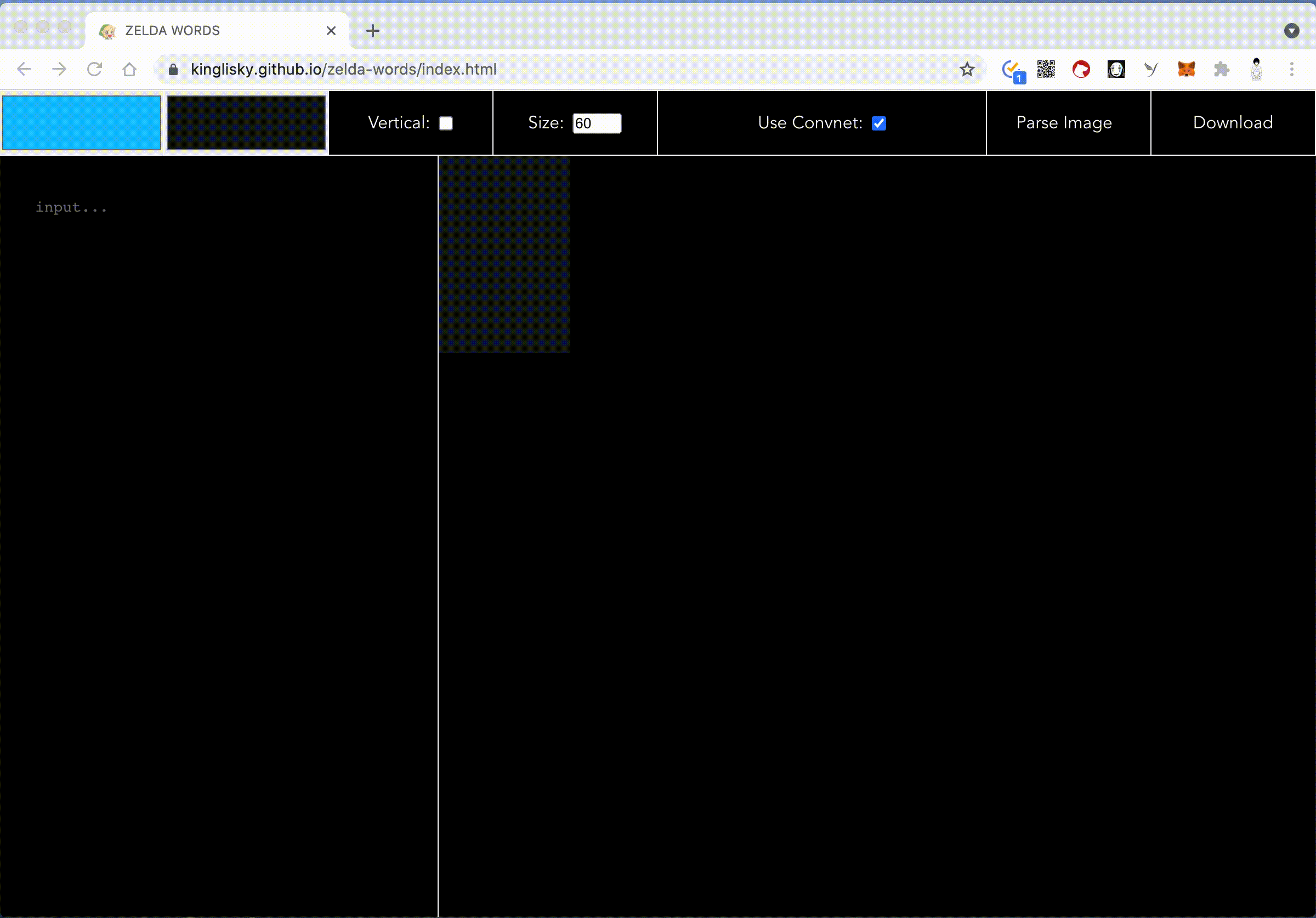## 如何画一只马

• 生成训练与测试数据
• 训练与评估模型
• 使用训练模型进行文字预测识别

## 生成训练数据

MNIST 数据集表示经过改进的 NIST 数据集。其中，NIST 是美国国家标准与技术研究所（National Institute of Standards and Technology）的简称，这是因为 NIST 数据集是由该研究所收集并整理完成的。M 表示“经过改进”的（modified），体现了MNIST数据集是在原 NIST 数据集基础之上所做的改进。MNIST 数据集主要改进了两个方面：第一，将图像标准化为统一的 28 像素 × 28 像素，并进行抗锯齿处理，这让训练集和测试集更加一致；第二，确保训练集和测试集的手写数字来自没有重合的两组参与者。这些改进让数据集变得更易用，并且能够更加客观地计算模型的准确率。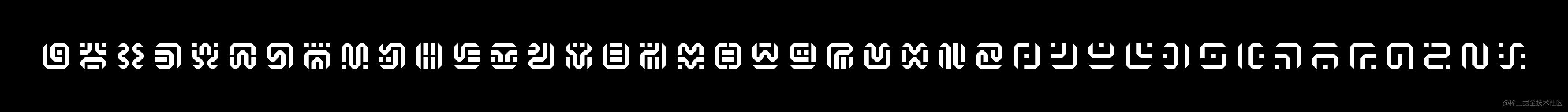### 张量与图片的 NHWC 编码

``````// 一维张量
[1, 0, 0]

// 二维张量
[
[1, 0, 0],
[0, 1, 0],
[0, 0, 1],
]

// 三维张量
[
[
[1, 0, 0],
[0, 1, 0],
[0, 0, 1],
],
[
[1, 0, 0],
[0, 1, 0],
[0, 0, 1],
],
[
[1, 0, 0],
[0, 1, 0],
[0, 0, 1],
]
]
// ... N 维张量
````````````[
// 第一行像素
[
[255, 255, 255], // rgb 通道值
[0, 0, 0],
],
// 第二行像素
[
[0, 0, 0],
[0, 0, 0],
],
]
``````

``````[
// 第一行像素
[
, // 只有一个灰度通道
,
],
// 第二行像素
[
,
,
],
]
``````

TensorFlow 有个张量形状的概念，简单来说就是用一个数组来描述张量每一维度的长度，有兴趣的同学可以看看张量的形状的定义。

``````[height, width, colorChannel]
``````

``````[
// 第一行像素
[
, // 只有一个灰度通道
,
],
// 第二行像素
[
,
,
],
]

// 转换成一维数组

[255, 0, 0, 0]
``````

``````[255, 0, 0, 0] // 图片 1（HWC）
[0, 255, 0, 0] // 图片 2（HWC）

[255, 0, 0, 0, 0, 255, 0, 0] // 组合成批次（NHWC）
``````

### 确认样本特征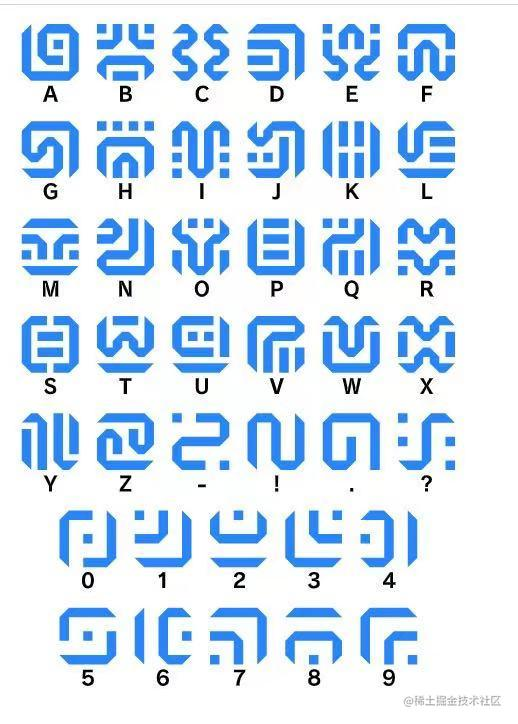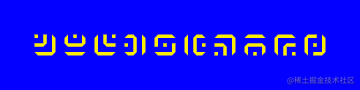• 图片文字不同
• 图片大小不同
• 图片的文字颜色不同
• 图片的背景颜色不同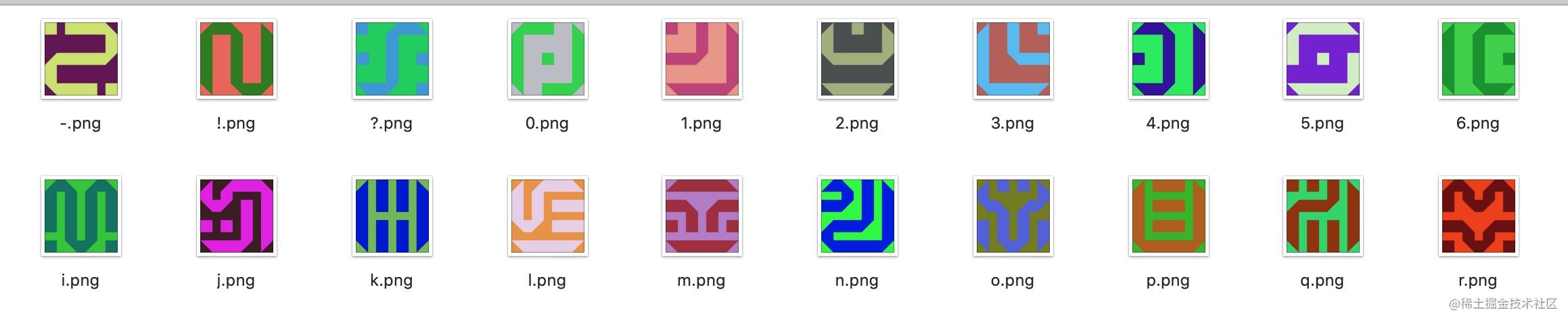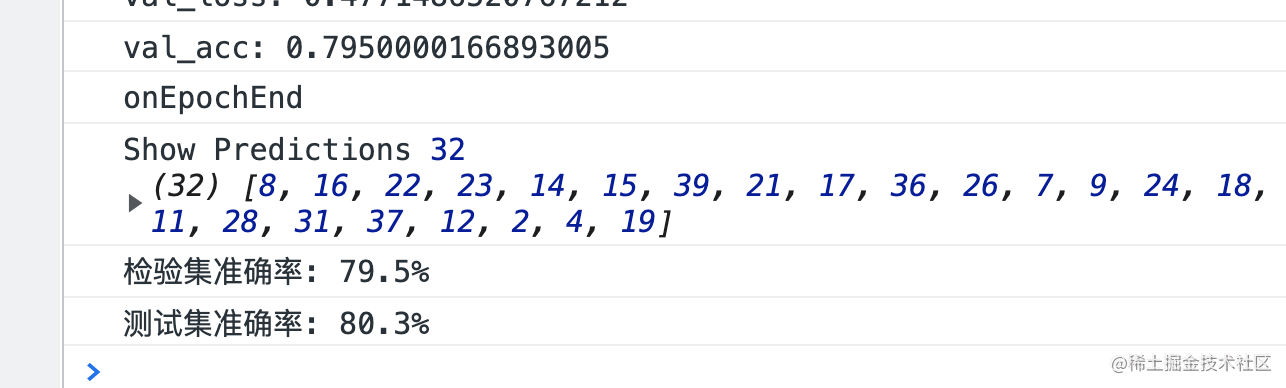• 图片的文字不同
• 图片的大小不同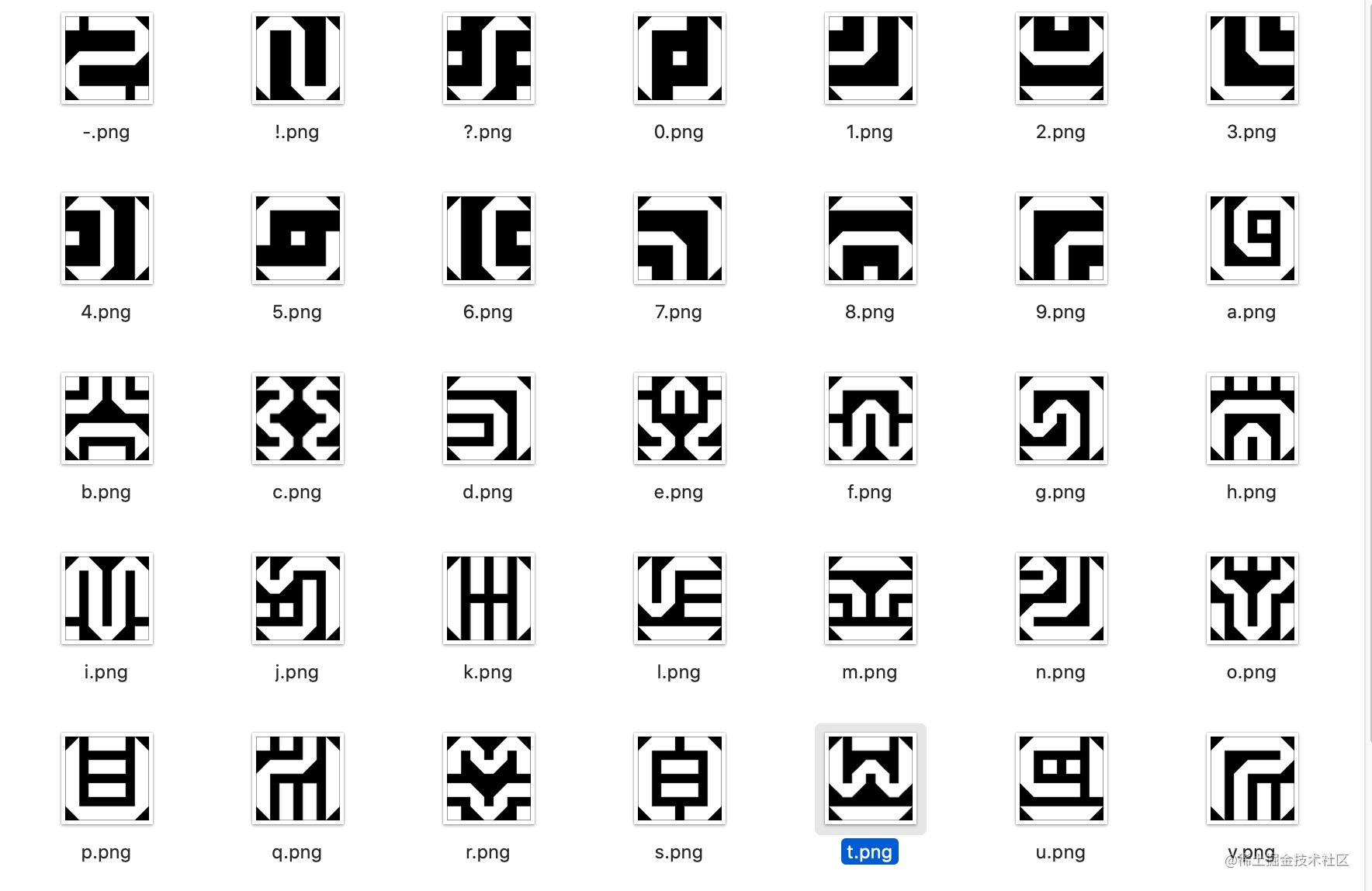### 生成训练图片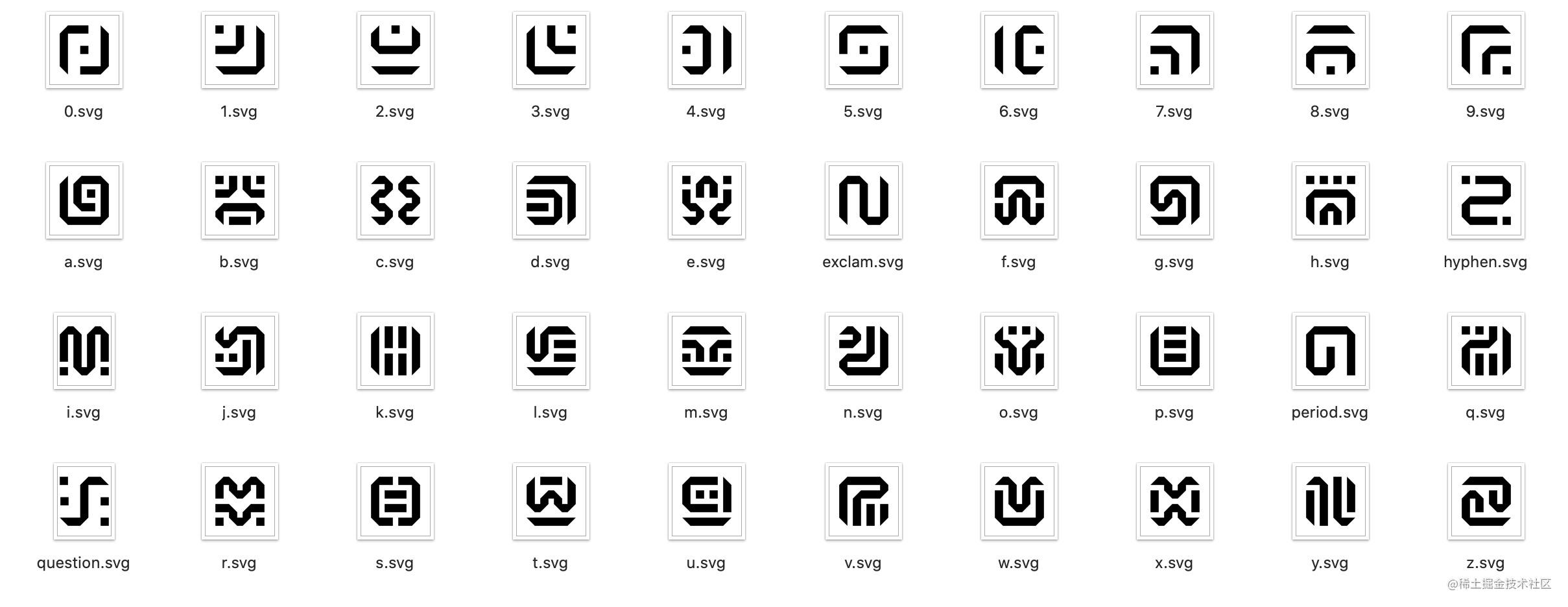``````// words.json
[
{
"symbol": "a",
"path": "sheikah-icon/a.svg"
},
{
"symbol": "b",
"path": "sheikah-icon/b.svg"
},
{
"symbol": "c",
"path": "sheikah-icon/c.svg"
},
......
]
``````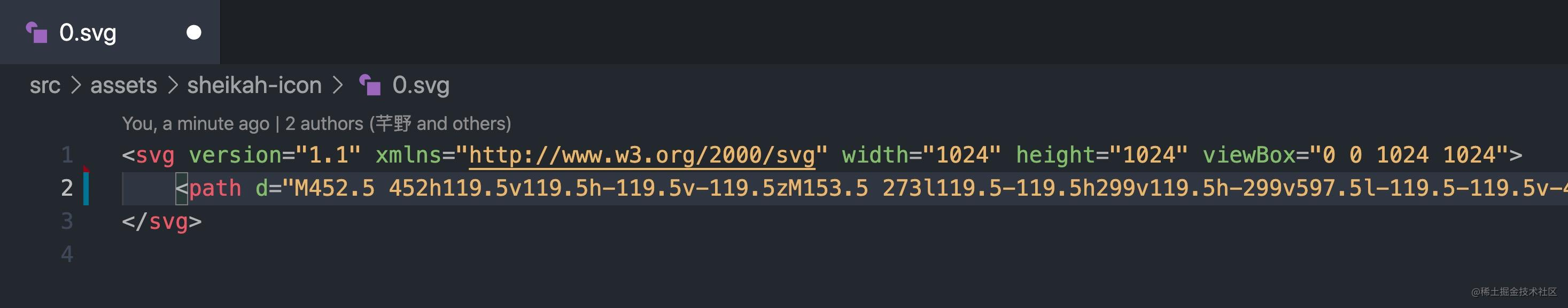``````function fillSvg(svg, color) {
const \$ = cheerio.load(svg, { xmlMode: true });
const fill = Color(color).hex();
\$('svg').attr('fill', fill);
return \$.xml();
}

const svgPath = path.join(__dirname, '../src/assets', word.path);
const data = await readFile(svgPath, 'utf8');
// 文字填充为白色
const svgContent = fillSvg(data, {
r: 255,
g: 255,
b: 255,
});
return Buffer.from(svgContent);
}
``````

``````const IMAGE_WIDTH = 28;
const IMAGE_HEIGHT = 28;

function randomValue(value, base = 0) {
return Math.floor(Math.random() * value + base);
}

async function createWordImage(word) {
const size = randomValue(200, 24);
// 生成大小不同的图片
const resizeImageBuffer = await sharp(svg)
.resize(size, size)
.trim()
.png().toBuffer();
// 统一缩放成 28 x 28 大小
const wordImageBuffer = await sharp(resizeImageBuffer)
.resize(IMAGE_WIDTH, IMAGE_HEIGHT)
.png().toBuffer();
// 字符背景图片
const baseImageBuffer = await sharp({
create: {
width: IMAGE_WIDTH,
height: IMAGE_HEIGHT,
channels: 4,
background: {
r: 0,
g: 0,
b: 0,
alpha: 0,
},
}
}).png().toBuffer();
// 将文字图片绘制到背景图片上，做锐化并且以 raw 格式输出
const image = await sharp(baseImageBuffer).composite([{
input: wordImageBuffer,
top: 0,
left: 0,
}]).sharpen().raw().toBuffer();
return image;
}
``````

sharp 中我们可以将图片导出成 buffer 来使用，最后一步我们将文字与背景图片融合后导出，导出前加了两个操作：

• sharpen 图片锐化抗锯齿处理
• raw 格式导出 buffer

``````const tf = require('@tensorflow/tfjs');
const WORDS = require('../src/data/words.json');
const WORDS_INDEXS = Array.from({ length: WORDS.length }).map((_, i) => i);
const IMAGE_WIDTH = 28;
const IMAGE_HEIGHT = 28;
const COUNT = 100;
const NAME = 'train';

(async function main() {
let data = null;
const indexs = [];
for (let i = 0; i < COUNT; i++) {
// 打乱字符 40 字符索引顺序
tf.util.shuffle(WORDS_INDEXS);
const createWords = WORDS_INDEXS.map(async (index) => {
const word = WORDS[index];
const buffer = await createWordImage(word);
return {
index,
buffer,
};
});
const res = await Promise.all(createWords);
res.forEach(({ index, buffer }) => {
const pixs = [];
// rgb 转灰度
for (let i = 0; i < buffer.length; i += 4) {
const a = buffer[i + 3] / 255;
const r = buffer[i] * a;
const g = buffer[i + 1] * a;
const b = buffer[i + 2] * a;
pixs.push(Math.floor(r * 0.299 + g * 0.587 + b * 0.114));
}
indexs.push(index);
const pixsBuffer = Buffer.from(pixs);
//  buffer 拼接图片
data = data ? Buffer.concat([data, pixsBuffer]) : pixsBuffer;
});
const meta = {
indexs,
count: (i + 1) * WORDS_INDEXS.length,
width: IMAGE_WIDTH,
height: IMAGE_HEIGHT,
buffer: `\${NAME}.buffer`,
};
// 保存训练数据
await writeFile(path.join(__dirname, `../src/data/\${NAME}.buffer`), data);
await writeFile(path.join(__dirname, `../src/data/\${NAME}.json`), JSON.stringify(meta));
}
console.log('done!');
})();
``````

## 训练与评估模型

### 加载数据与 oneHot 编码

``````const loadBuffer = async (data) => {
const {
count,
width,
height,
url,
indexs,
} = data;
const buffers = await fetch(url).then(res => res.arrayBuffer());
const images = new Float32Array(new Uint8ClampedArray(buffers));
for (let i = 0; i < images.length; i++) {
// 将像素值统一成 0 ~ 1 Float32 值
images[i] = images[i] / 255;
}
const labels = new Int32Array(indexs);
return {
count,
width,
height,
images,
labels,
};
}
``````

• 黑洞体积（1 ~ 1000）
• 黑洞质量（100000000 ~ 100000000000）

• 黑洞体积（0 ~ 1）
• 黑洞质量（0 ~ 1）

``````// data.js
import * as tf from '@tensorflow/tfjs';
import TRAIN from '../data/train.json';
import TEST from '../data/test.json';
import trainBufferURL from '../data/train.buffer?url';
import testBufferURL from '../data/test.buffer?url';

TRAIN.url = trainBufferURL;
TEST.url = testBufferURL;

export const WORDS_COUNT = 40;
export const IMAGE_H = 28;
export const IMAGE_W = 28;

class Dataset {
constructor() {
this.dataset = {};
}

this.dataset = {
train,
test,
};
}

getData(key) {
const target = this.dataset[key];
const imagesShape = [target.count, target.height, target.height, 1];
return {
// 转成 [8000, 28, 28, 1] 的张量
images: tf.tensor4d(target.images, imagesShape),
// 输出目标这里使用 oneHot 编码
labels: tf.oneHot(tf.tensor1d(target.labels, 'int32'), WORDS_COUNT).toFloat(),
};
}

// 获取训练数据
getTrainData() {
return this.getData('train');
}

// 获取测试数据
getTestData(numExamples) {
const res = this.getData('test');
if (numExamples) {
return {
images: res.images.slice([0, 0, 0, 0], [numExamples, IMAGE_H, IMAGE_W, 1]),
labels: res.labels.slice([0, 0], [numExamples, WORDS_COUNT]),
};
}
return res;
}
}

export const dataset = new Dataset();
``````

``````const labels = tf.oneHot(tf.tensor1d([0, 1, 2], 'int32'), 3);
console.log(labels, labels.dataSync());
``````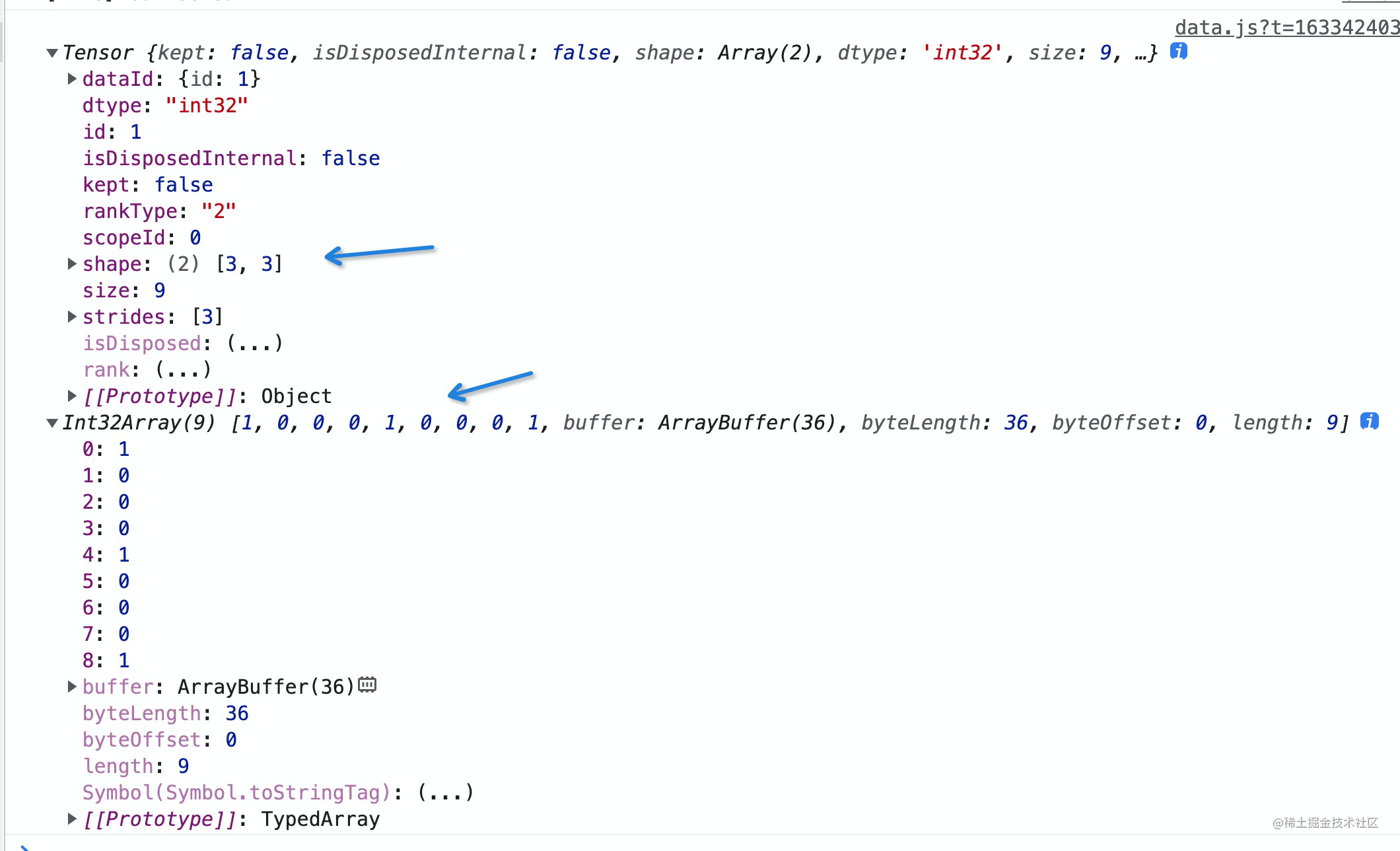``````[0, 1, 2]
// 原本的 [N] 形状的数据变成了 [N, 3] 形状
[
[1, 0, 0],
[0, 1, 0],
[0 0, 1],
]
``````
• A 索引为 0 对应编码 [1, 0, 0]
• B 索引为 1 对应编码 [0, 1, 0]
• C 索引为 2 对应编码 [0, 0, 1]

``````[
// 001 -> C
[
0.022, // 0
0.024, // 0
0.026, // 1
],
// 010 -> B
[
0.021, // 0
0.028, // 1
0.026, // 0
],
// 100 -> A
[
0.027, // 1
0.024, // 0
0.025, // 0
],
]
``````

### 训练模型

``````import * as tf from '@tensorflow/tfjs';
import { IMAGE_H, IMAGE_W, WORDS_COUNT } from './data';

function createModel() {
const model = tf.sequential();
// conv2d 层，进行卷积操作
inputShape: [IMAGE_H, IMAGE_W, 1],
kernelSize: 3,
filters: 16,
activation: 'relu'
}));
// 卷积后进行池化
model.add(tf.layers.maxPooling2d({ poolSize: 2, strides: 2 }));
// 在重复一次卷积与池化
model.add(tf.layers.conv2d({ kernelSize: 3, filters: 32, activation: 'relu' }));
model.add(tf.layers.maxPooling2d({ poolSize: 2, strides: 2 }));
model.add(tf.layers.conv2d({ kernelSize: 3, filters: 32, activation: 'relu' }));
// 扁平化张量
// 添加层密集增加神经网络的容量
model.add(tf.layers.dense({ units: 64, activation: 'relu' }));
// 为多分类问题配置归一化指数激活函数
model.add(tf.layers.dense({ units: WORDS_COUNT, activation: 'softmax' }));
return model;
}
``````

``````tf.layers.dense({ units: WORDS_COUNT, activation: 'softmax' });
``````

``````import * as tf from '@tensorflow/tfjs';
import { dataset, IMAGE_H, IMAGE_W, WORDS_COUNT } from './data';

async function train({ model, data }) {
model.compile({
// RMSprop 优化器
optimizer: 'rmsprop',
// 交叉熵损失函数
loss: 'categoricalCrossentropy',
// 度量依据精度
metrics: ['accuracy'],
});
// 每个批次选多少个样本
const batchSize = 400;
// 校验集比例
const validationSplit = 0.15;
// 训练轮次
const trainEpochs = 20;

let trainBatchCount = 0;
let trainEpochCount = 0;

const trainData = data.getTrainData();
const testData = data.getTestData();
// 剔除掉校验集合后的总训练样本数量
const totalNumBatches = Math.ceil(trainData.images.shape * (1 - validationSplit) / batchSize) * trainEpochs;

let valAcc;
// 执行模型训练
await model.fit(trainData.images, trainData.labels, {
batchSize,
validationSplit,
epochs: trainEpochs,
callbacks: {
// 每个批次训练结束调用
onBatchEnd: async (batch, logs) => {
trainBatchCount++;
console.log(`训练进度：\${(trainBatchCount / totalNumBatches * 100).toFixed(1)}%`);
console.log(`损失: \${logs.loss}`);
console.log(`准确率: \${logs.acc}`);
await tf.nextFrame();
},
// 每个轮次训练结束调用
onEpochEnd: async (epoch, logs) => {
trainEpochCount++;
valAcc = logs.val_acc;
console.log(`训练进度：轮次 \${trainEpochCount}`);
console.log(`校验集损失: \${logs.val_loss}`);
console.log(`校验集准确率: \${logs.val_acc}`);
await tf.nextFrame();
}
}
});
const testResult = model.evaluate(testData.images, testData.labels);
const testAccPercent = testResult.dataSync() * 100;
const finalValAccPercent = valAcc * 100;
console.log(`检验集准确率: \${finalValAccPercent.toFixed(1)}%`);
console.log(`测试集准确率: \${testAccPercent.toFixed(1)}%`);
console.log('保存模型', saveResults);
}

async function run() {
const model = createModel();
train({
model,
data: dataset,
});
};
``````

• `batchSize` 每个训练批次样本数量
• `validationSplit` 训练时校验集比例
• `trainEpochs` 训练次数（轮次）

`validationSplit` 表示需要从训练集合取多大比例的样本数据用做校验，我们训练集有 8000 个样本，validationSplit 为 0.15，`8000 * 0.15 = 1200` 则每次训练我们从训练集中单独调出 1200 个样本用做校验，校验集的数据不会出现在训练中，每个轮次训练结束我们可以使用校验集数据对模型做一个评估，判断模式的可用性。

`trainEpochs` 训练的次数（轮数）会显著影响模型最终准确性，这也是最简单好用的提升模型质量的方法了，我们这里尝试了训练 1 次、5 次、10 次与 18 次的效果：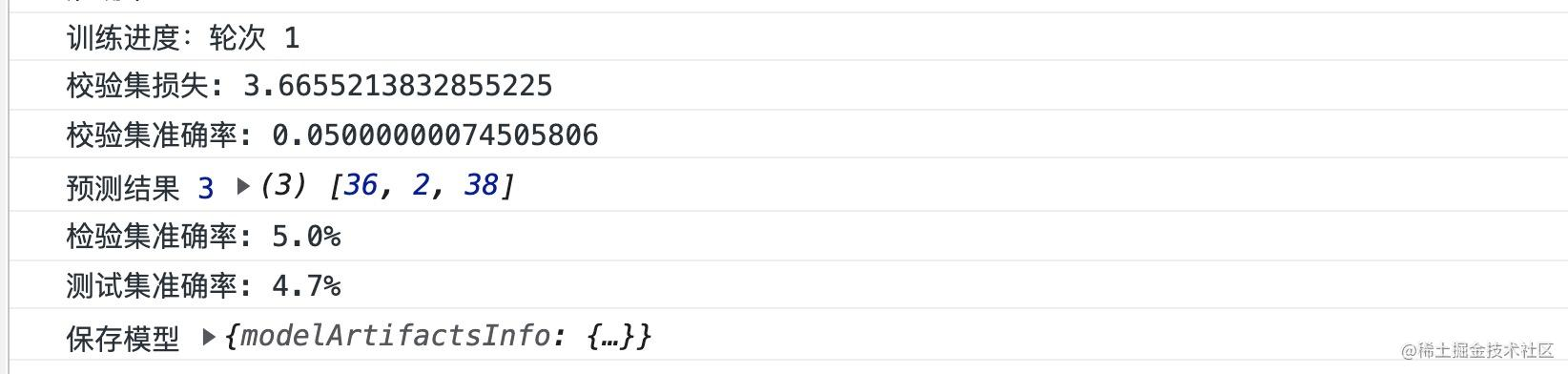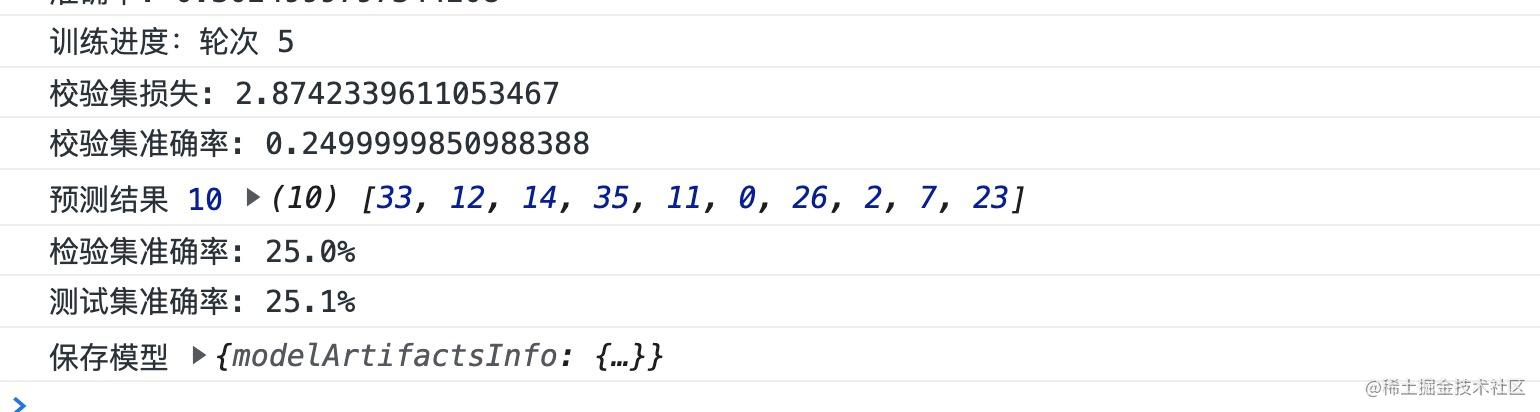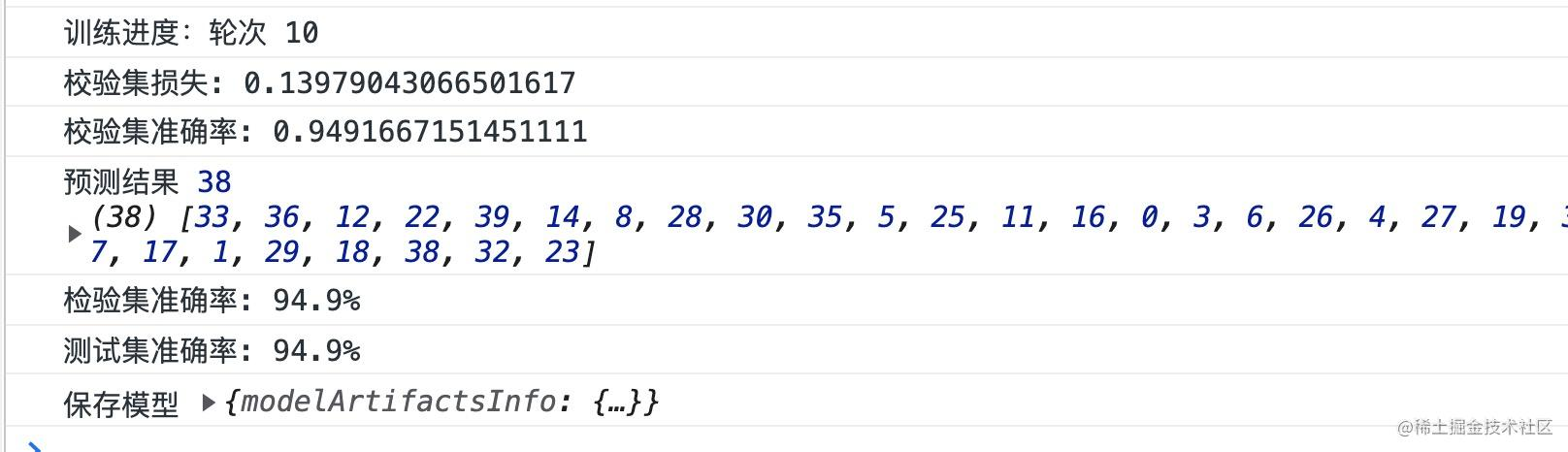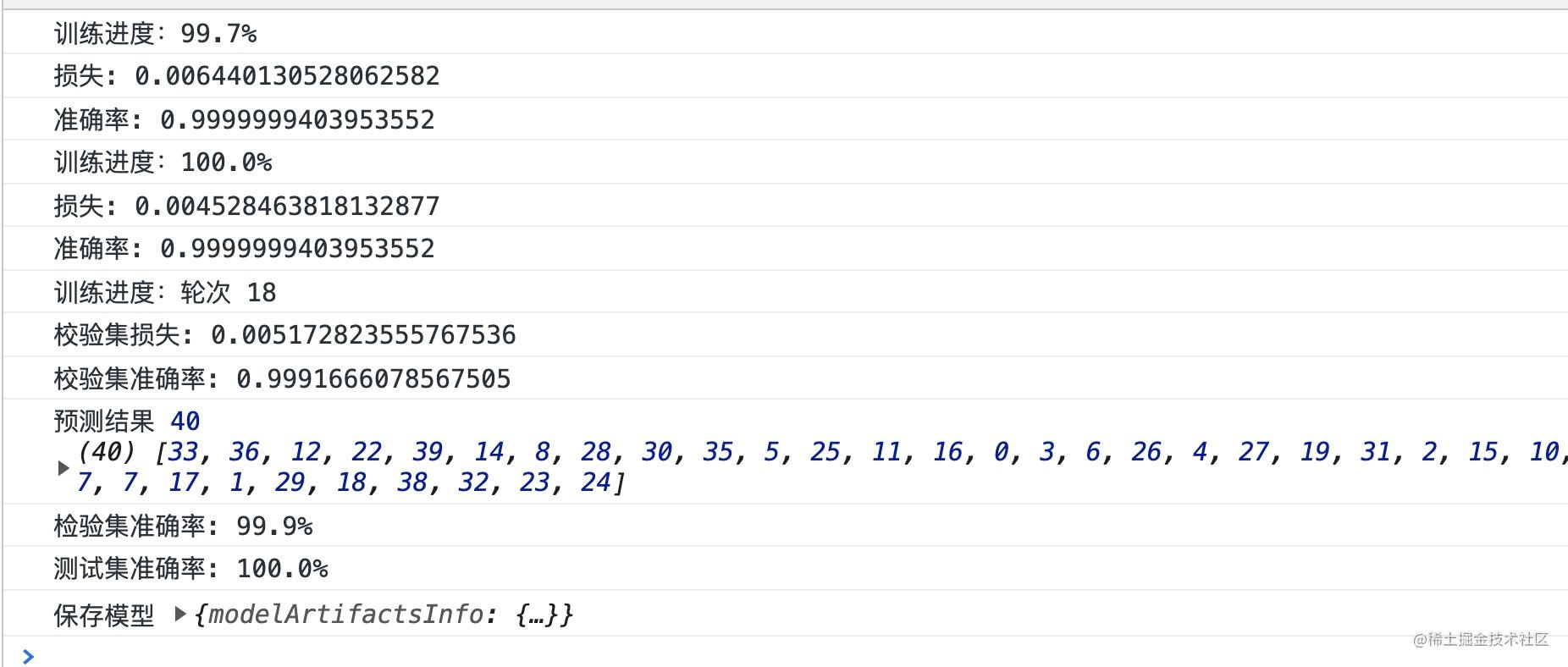## 模型的导出使用

``````await model.save('downloads://zelda-words-model');
``````

• model.json 是一个 JSON 文件，它包含了保存的模型拓扑结构。此处的“拓扑结构”包括：组成模型的层的类型、各层对应的配置参数，以及层之间的连接方式。
• 除了模型的拓扑结构，model.json 还包含模型的权重清单。权重清单部分包含模型所有权重的名字、形状、数据类型，以及权重值存储的位置。weights.bin是一个二进制文件，它存储了模型的所有权重值。

``````import * as tf from '@tensorflow/tfjs';
import { dataset } from './data';
import modelURL from '../data/model.json?url';

async function predict() {
// 载入训练完成的模型
const examples = dataset.getTestData(40);
// 使用模型进行预测
const output = model.predict(examples.images);
const axis = 1;
// 输出测试集目标索引
const labels = Array.from(examples.labels.argMax(axis).dataSync());
// 预测结果的索引
const predictions = Array.from(output.argMax(axis).dataSync());
const res = predictions.filter((it, index) => it === labels[index]);
console.log('预测结果', res.length, res);
}
``````

``````[
[0.01, 0.02, 0.03],
[0.03, 0.02, 0.01],
[0.01, 0.03, 0.03],
];

// -- argMax(1) -->

[
2,
0,
1,
];
``````

``````function convertToPredictData(images: Chunk[], imageSize: number) {
images.forEach(it => {
const imageData = resizeCanvas(it.canvas, imageSize);
const pixs = new Float32Array(imageData.data.length / 4);
let index = 0;
// rgb 转灰度
for (let i = 0; i < imageData.data.length; i += 4) {
const r = imageData.data[i];
const g = imageData.data[i + 1];
const b = imageData.data[i + 2];
pixs[index] = (r * 0.299 + g * 0.587 + b * 0.114) / 255;
index += 1;
}
it.data = pixs;
});
const shape: [number, number, number, number] = [images.length, imageSize, imageSize, 1];
const shapeSize = tf.util.sizeFromShape(shape);
const concatData = new Float32Array(shapeSize);
images.forEach((image, index) => {
concatData.set(image.data as Float32Array, index * imageSize * imageSize);
});
// 将图片转换成张量
return tf.tensor4d(concatData, shape);
}

export async function readMetaInfoByCnn(imageUrl: string) {
const modelURL = 'https://xxx-server.com/model.json';
const imageSize = 28;
// 将希卡文的图片拆分出来
// 转换成模型需要的张量格式
const predictData = convertToPredictData(images, imageSize);
// 加载训练号的模型
const output = model.predict(predictData) as tf.Tensor;
const axis = 1;
// 获取预测结果的索引
const predictIndexs = Array.from(output.argMax(axis).dataSync());
// 通过索引找到目标字符
const results = predictIndexs.map((predictIndex, index) => {
const target = words[predictIndex];
return {
...images[index],
word: target.symbol,
};

});
console.log('results', results);
if (results.length) {
return printfSymbols(
results,
);
}
throw new Error('PARSE ERROR');
}
``````

## 最后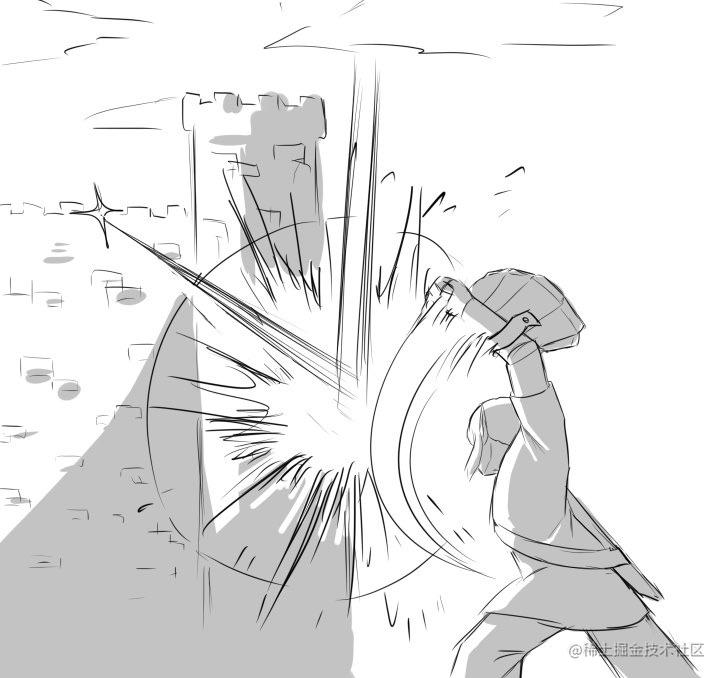2 回复

lz厉害，收藏了，慢慢看